Fraction Rules - Definition with Examples

The Complete K-5 Math Learning Program Built for Your Child

• 30 Million Kids

Loved by kids and parent worldwide

• 50,000 Schools

Trusted by teachers across schools

• Comprehensive Curriculum

Aligned to Common Core

What is Fraction Rules?

Fraction: A fraction is a part of a whole or a collection and it consists of a numerator and denominator.

Example: If we serve1 part of a cake with 8 equal parts, we have served 18 of the cake.

Let us see how to solve operations involving fractions.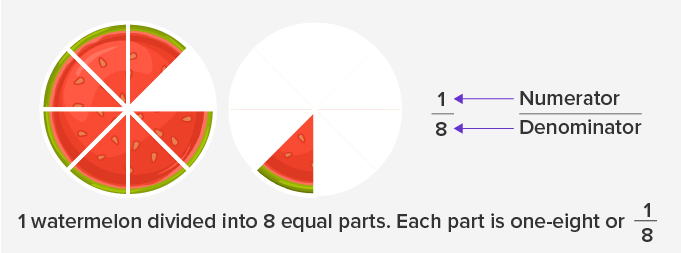Adding or subtracting fractions with the same denominator

While adding or subtracting two fractions; we need to make sure that the denominators are the same.

Steps:

• Add or subtract the numerators.

• Keep the denominator the same.

• Reduce the answer, if possible.

Example: Solve  114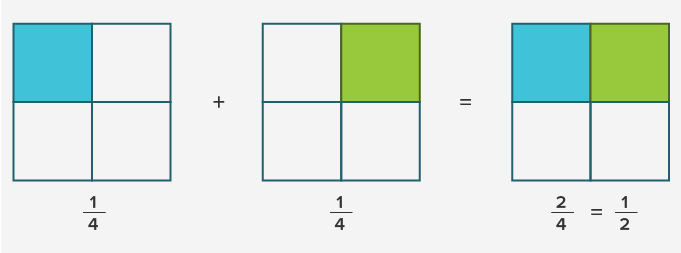Example: Subtract 14 from 34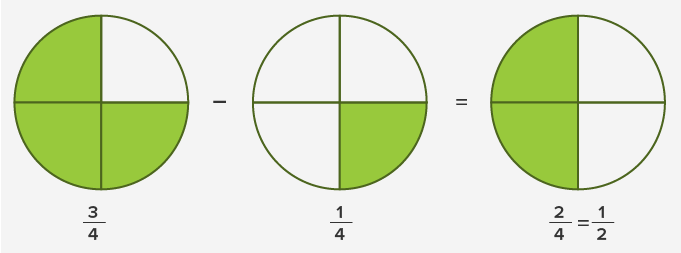Adding or subtracting fractions with the different denominators:

If the denominators are not the same:

• First, make them the same

• Then add or subtract like fractions with the same denominators.

Example: To solve 14 + 12 , we first make the denominators the same.

We change the denominator 2 and make it 4 by multiplying it by 2. However, we need to multiply the numerator and denominator both by 2 to keep the value of the fraction unchanged.

Multiplying 12 ✕ 22 = 24

Since the denominators are the same we can now add both the fractions.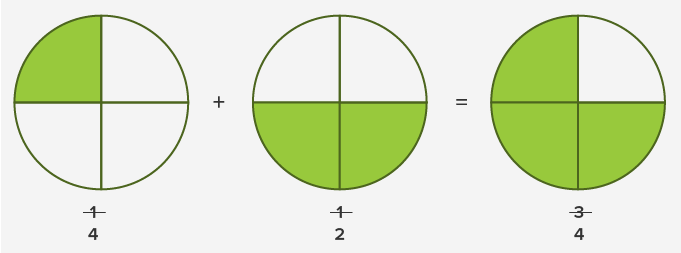Similarly, we use these rules for subtraction.

Multiplying Fractions

To multiply two fractions we simply multiply the numerators and denominators.

Example:

23 ✕ 315 = ?

First, simplify the fraction 315 to its lowest term.Dividing Fractions

While dividing two fractions:

• Inverse the second fraction, that is, interchange its numerator and denominator to get the reciprocal.

• Multiply the first fraction by the reciprocal of the second fraction.

Example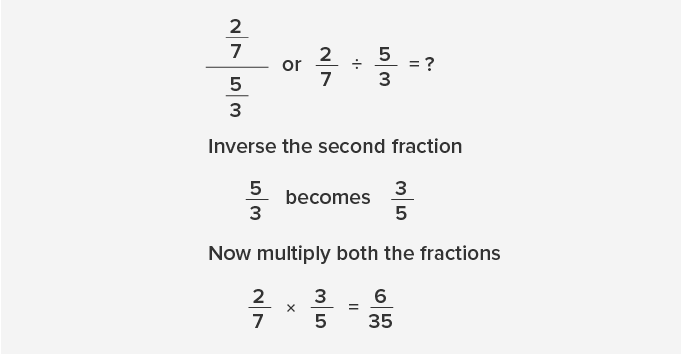Solving improper fractions:

Fractions with a numerator larger than the denominator are called improper fractions. When we solve improper fractions, the result can be a mixed number (a fraction with a whole number and a proper fraction).

Example

38= ?

• Divide the numerator by the denominator.

38 ÷ 7 = 5 Quotient and 3 Remainder

• Write down the whole number answer.

5

• Then write down the remainder above the denominator.

5 37

Therefore,  387 = 5 37

Thus, by solving an improper fraction 387 we get a mixed number 5 37

 Fun Facts We can multiply two fractions with different denominators, but we cannot add and subtract them.

Won Numerous Awards & Honors